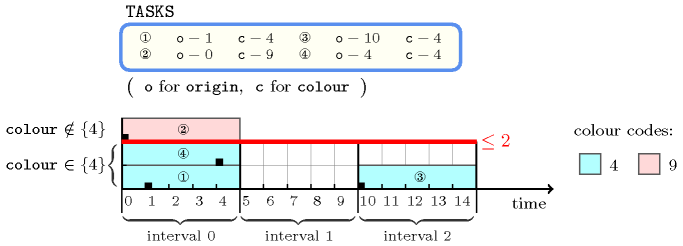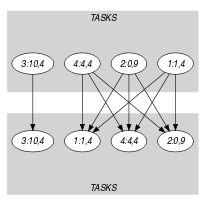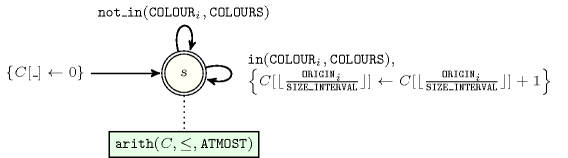## 5.197. interval_and_count

Origin
Constraint

$\mathrm{𝚒𝚗𝚝𝚎𝚛𝚟𝚊𝚕}_\mathrm{𝚊𝚗𝚍}_\mathrm{𝚌𝚘𝚞𝚗𝚝}\left(\mathrm{𝙰𝚃𝙼𝙾𝚂𝚃},\mathrm{𝙲𝙾𝙻𝙾𝚄𝚁𝚂},\mathrm{𝚃𝙰𝚂𝙺𝚂},\mathrm{𝚂𝙸𝚉𝙴}_\mathrm{𝙸𝙽𝚃𝙴𝚁𝚅𝙰𝙻}\right)$

Arguments
 $\mathrm{𝙰𝚃𝙼𝙾𝚂𝚃}$ $\mathrm{𝚒𝚗𝚝}$ $\mathrm{𝙲𝙾𝙻𝙾𝚄𝚁𝚂}$ $\mathrm{𝚌𝚘𝚕𝚕𝚎𝚌𝚝𝚒𝚘𝚗}\left(\mathrm{𝚟𝚊𝚕}-\mathrm{𝚒𝚗𝚝}\right)$ $\mathrm{𝚃𝙰𝚂𝙺𝚂}$ $\mathrm{𝚌𝚘𝚕𝚕𝚎𝚌𝚝𝚒𝚘𝚗}\left(\mathrm{𝚘𝚛𝚒𝚐𝚒𝚗}-\mathrm{𝚍𝚟𝚊𝚛},\mathrm{𝚌𝚘𝚕𝚘𝚞𝚛}-\mathrm{𝚍𝚟𝚊𝚛}\right)$ $\mathrm{𝚂𝙸𝚉𝙴}_\mathrm{𝙸𝙽𝚃𝙴𝚁𝚅𝙰𝙻}$ $\mathrm{𝚒𝚗𝚝}$
Restrictions
 $\mathrm{𝙰𝚃𝙼𝙾𝚂𝚃}\ge 0$ $\mathrm{𝚛𝚎𝚚𝚞𝚒𝚛𝚎𝚍}$$\left(\mathrm{𝙲𝙾𝙻𝙾𝚄𝚁𝚂},\mathrm{𝚟𝚊𝚕}\right)$ $\mathrm{𝚍𝚒𝚜𝚝𝚒𝚗𝚌𝚝}$$\left(\mathrm{𝙲𝙾𝙻𝙾𝚄𝚁𝚂},\mathrm{𝚟𝚊𝚕}\right)$ $\mathrm{𝚛𝚎𝚚𝚞𝚒𝚛𝚎𝚍}$$\left(\mathrm{𝚃𝙰𝚂𝙺𝚂},\left[\mathrm{𝚘𝚛𝚒𝚐𝚒𝚗},\mathrm{𝚌𝚘𝚕𝚘𝚞𝚛}\right]\right)$ $\mathrm{𝚃𝙰𝚂𝙺𝚂}.\mathrm{𝚘𝚛𝚒𝚐𝚒𝚗}\ge 0$ $\mathrm{𝚂𝙸𝚉𝙴}_\mathrm{𝙸𝙽𝚃𝙴𝚁𝚅𝙰𝙻}>0$
Purpose

First consider the set of tasks of the $\mathrm{𝚃𝙰𝚂𝙺𝚂}$ collection, where each task has a specific colour that may not be initially fixed. Then consider the intervals of the form $\left[k·\mathrm{𝚂𝙸𝚉𝙴}_\mathrm{𝙸𝙽𝚃𝙴𝚁𝚅𝙰𝙻},k·\mathrm{𝚂𝙸𝚉𝙴}_\mathrm{𝙸𝙽𝚃𝙴𝚁𝚅𝙰𝙻}+\mathrm{𝚂𝙸𝚉𝙴}_\mathrm{𝙸𝙽𝚃𝙴𝚁𝚅𝙰𝙻}-1\right]$, where $k$ is an integer. The $\mathrm{𝚒𝚗𝚝𝚎𝚛𝚟𝚊𝚕}_\mathrm{𝚊𝚗𝚍}_\mathrm{𝚌𝚘𝚞𝚗𝚝}$ constraint forces that, for each interval ${I}_{k}$ previously defined, the total number of tasks, which both are assigned to ${I}_{k}$ and take their colour in $\mathrm{𝙲𝙾𝙻𝙾𝚄𝚁𝚂}$, does not exceed the limit $\mathrm{𝙰𝚃𝙼𝙾𝚂𝚃}$.

Example
$\left(\begin{array}{c}2,〈4〉,\hfill \\ 〈\begin{array}{cc}\mathrm{𝚘𝚛𝚒𝚐𝚒𝚗}-1\hfill & \mathrm{𝚌𝚘𝚕𝚘𝚞𝚛}-4,\hfill \\ \mathrm{𝚘𝚛𝚒𝚐𝚒𝚗}-0\hfill & \mathrm{𝚌𝚘𝚕𝚘𝚞𝚛}-9,\hfill \\ \mathrm{𝚘𝚛𝚒𝚐𝚒𝚗}-10\hfill & \mathrm{𝚌𝚘𝚕𝚘𝚞𝚛}-4,\hfill \\ \mathrm{𝚘𝚛𝚒𝚐𝚒𝚗}-4\hfill & \mathrm{𝚌𝚘𝚕𝚘𝚞𝚛}-4\hfill \end{array}〉,5\hfill \end{array}\right)$

Figure 5.197.1 shows the solution associated with the example. The constraint $\mathrm{𝚒𝚗𝚝𝚎𝚛𝚟𝚊𝚕}_\mathrm{𝚊𝚗𝚍}_\mathrm{𝚌𝚘𝚞𝚗𝚝}$ holds since, for each interval, the number of tasks taking colour 4 does not exceed the limit 2.

##### Figure 5.197.1. The $\mathrm{𝚒𝚗𝚝𝚎𝚛𝚟𝚊𝚕}_\mathrm{𝚊𝚗𝚍}_\mathrm{𝚌𝚘𝚞𝚗𝚝}$ solution to the Example slot with the use of each intervalTypical
 $\mathrm{𝙰𝚃𝙼𝙾𝚂𝚃}>0$ $\mathrm{𝙰𝚃𝙼𝙾𝚂𝚃}<|\mathrm{𝚃𝙰𝚂𝙺𝚂}|$ $|\mathrm{𝙲𝙾𝙻𝙾𝚄𝚁𝚂}|>0$ $|\mathrm{𝚃𝙰𝚂𝙺𝚂}|>1$ $\mathrm{𝚛𝚊𝚗𝚐𝚎}$$\left(\mathrm{𝚃𝙰𝚂𝙺𝚂}.\mathrm{𝚘𝚛𝚒𝚐𝚒𝚗}\right)>1$ $\mathrm{𝚛𝚊𝚗𝚐𝚎}$$\left(\mathrm{𝚃𝙰𝚂𝙺𝚂}.\mathrm{𝚌𝚘𝚕𝚘𝚞𝚛}\right)>1$ $\mathrm{𝚂𝙸𝚉𝙴}_\mathrm{𝙸𝙽𝚃𝙴𝚁𝚅𝙰𝙻}>1$
Symmetries
• $\mathrm{𝙰𝚃𝙼𝙾𝚂𝚃}$ can be increased.

• Items of $\mathrm{𝙲𝙾𝙻𝙾𝚄𝚁𝚂}$ are permutable.

• Items of $\mathrm{𝚃𝙰𝚂𝙺𝚂}$ are permutable.

• One and the same constant can be added to the $\mathrm{𝚘𝚛𝚒𝚐𝚒𝚗}$ attribute of all items of $\mathrm{𝚃𝙰𝚂𝙺𝚂}$.

• An occurrence of a value of $\mathrm{𝚃𝙰𝚂𝙺𝚂}.\mathrm{𝚘𝚛𝚒𝚐𝚒𝚗}$ that belongs to the $k$-th interval, of size $\mathrm{𝚂𝙸𝚉𝙴}_\mathrm{𝙸𝙽𝚃𝙴𝚁𝚅𝙰𝙻}$, can be replaced by any other value of the same interval.

• An occurrence of a value of $\mathrm{𝚃𝙰𝚂𝙺𝚂}.\mathrm{𝚌𝚘𝚕𝚘𝚞𝚛}$ that belongs to $\mathrm{𝙲𝙾𝙻𝙾𝚄𝚁𝚂}.\mathrm{𝚟𝚊𝚕}$ (resp. does not belong to $\mathrm{𝙲𝙾𝙻𝙾𝚄𝚁𝚂}.\mathrm{𝚟𝚊𝚕}$) can be replaced by any other value in $\mathrm{𝙲𝙾𝙻𝙾𝚄𝚁𝚂}.\mathrm{𝚟𝚊𝚕}$ (resp. not in $\mathrm{𝙲𝙾𝙻𝙾𝚄𝚁𝚂}.\mathrm{𝚟𝚊𝚕}$).

Arg. properties
• Contractible wrt. $\mathrm{𝙲𝙾𝙻𝙾𝚄𝚁𝚂}$.

• Contractible wrt. $\mathrm{𝚃𝙰𝚂𝙺𝚂}$.

Usage

This constraint was originally proposed for dealing with timetabling problems. In this context the different intervals are interpreted as morning and afternoon periods of different consecutive days. Each colour corresponds to a type of course (i.e., French, mathematics). There is a restriction on the maximum number of courses of a given type each morning as well as each afternoon.

Remark

If we want to only consider intervals that correspond to the morning or to the afternoon we could extend the $\mathrm{𝚒𝚗𝚝𝚎𝚛𝚟𝚊𝚕}_\mathrm{𝚊𝚗𝚍}_\mathrm{𝚌𝚘𝚞𝚗𝚝}$ constraint in the following way:

• We introduce two extra parameters $\mathrm{𝚁𝙴𝚂𝚃}$ and $\mathrm{𝚀𝚄𝙾𝚃𝙸𝙴𝙽𝚃}$ that correspond to non-negative integers such that $\mathrm{𝚁𝙴𝚂𝚃}$ is strictly less than $\mathrm{𝚀𝚄𝙾𝚃𝙸𝙴𝙽𝚃}$,

• We add the following condition to the arc constraint: $\left(\mathrm{𝚝𝚊𝚜𝚔𝚜}\mathtt{1}.\mathrm{𝚘𝚛𝚒𝚐𝚒𝚗}/\mathrm{𝚂𝙸𝚉𝙴}_\mathrm{𝙸𝙽𝚃𝙴𝚁𝚅𝙰𝙻}\right)\equiv \mathrm{𝚁𝙴𝚂𝚃}\left(\mathrm{mod}\mathrm{𝚀𝚄𝙾𝚃𝙸𝙴𝙽𝚃}\right)$

Now, if we want to express a constraint on the morning intervals, we set $\mathrm{𝚁𝙴𝚂𝚃}$ to 0 and $\mathrm{𝚀𝚄𝙾𝚃𝙸𝙴𝙽𝚃}$ to 2.

Reformulation

Let $K$ denote the index of the last possible interval where the tasks can be assigned: $K=⌊\frac{{max}_{i\in \left[1,|\mathrm{𝚃𝙰𝚂𝙺𝚂}|\right]}\left(\overline{\mathrm{𝚃𝙰𝚂𝙺𝚂}\left[i\right].\mathrm{𝚘𝚛𝚒𝚐𝚒𝚗}}\right)+\mathrm{𝚂𝙸𝚉𝙴}_\mathrm{𝙸𝙽𝚃𝙴𝚁𝚅𝙰𝙻}-1}{\mathrm{𝚂𝙸𝚉𝙴}_\mathrm{𝙸𝙽𝚃𝙴𝚁𝚅𝙰𝙻}}⌋$. The $\mathrm{𝚒𝚗𝚝𝚎𝚛𝚟𝚊𝚕}_\mathrm{𝚊𝚗𝚍}_\mathrm{𝚌𝚘𝚞𝚗𝚝}$$\left(\mathrm{𝙰𝚃𝙼𝙾𝚂𝚃},\mathrm{𝙲𝙾𝙻𝙾𝚄𝚁𝚂},\mathrm{𝚃𝙰𝚂𝙺𝚂},\mathrm{𝚂𝙸𝚉𝙴}_\mathrm{𝙸𝙽𝚃𝙴𝚁𝚅𝙰𝙻}\right)$ constraint can be expressed in term of a set of reified constraints and of $K$ arithmetic constraints (i.e., $\mathrm{𝚜𝚞𝚖}_\mathrm{𝚌𝚝𝚛}$ constraints).

1. For each task $\mathrm{𝚃𝙰𝚂𝙺𝚂}\left[i\right]$ $\left(i\in \left[1,|\mathrm{𝚃𝙰𝚂𝙺𝚂}|\right]\right)$ of the $\mathrm{𝚃𝙰𝚂𝙺𝚂}$ collection we create a 0-1 variable ${B}_{i}$ that will be set to 1 if and only if task $\mathrm{𝚃𝙰𝚂𝙺𝚂}\left[i\right]$ takes a colour within the set of colours $\mathrm{𝙲𝙾𝙻𝙾𝚄𝚁𝚂}$:

${B}_{i}⇔\mathrm{𝚃𝙰𝚂𝙺𝚂}\left[i\right].\mathrm{𝚌𝚘𝚕𝚘𝚞𝚛}=\mathrm{𝙲𝙾𝙻𝙾𝚄𝚁𝚂}\left[1\right].\mathrm{𝚟𝚊𝚕}\vee$

$\mathrm{𝚃𝙰𝚂𝙺𝚂}\left[i\right].\mathrm{𝚌𝚘𝚕𝚘𝚞𝚛}=\mathrm{𝙲𝙾𝙻𝙾𝚄𝚁𝚂}\left[2\right].\mathrm{𝚟𝚊𝚕}\vee$

$\cdots \cdots \cdots \cdots \cdots \cdots \cdots \cdots \cdots \cdots$

$\mathrm{𝚃𝙰𝚂𝙺𝚂}\left[i\right].\mathrm{𝚌𝚘𝚕𝚘𝚞𝚛}=\mathrm{𝙲𝙾𝙻𝙾𝚄𝚁𝚂}\left[|\mathrm{𝙲𝙾𝙻𝙾𝚄𝚁𝚂}|\right].\mathrm{𝚟𝚊𝚕}$.

2. For each task $\mathrm{𝚃𝙰𝚂𝙺𝚂}\left[i\right]$ $\left(i\in \left[1,|\mathrm{𝚃𝙰𝚂𝙺𝚂}|\right]\right)$ and for each interval $\left[k·\mathrm{𝚂𝙸𝚉𝙴}_\mathrm{𝙸𝙽𝚃𝙴𝚁𝚅𝙰𝙻},k·\mathrm{𝚂𝙸𝚉𝙴}_\mathrm{𝙸𝙽𝚃𝙴𝚁𝚅𝙰𝙻}+\mathrm{𝚂𝙸𝚉𝙴}_\mathrm{𝙸𝙽𝚃𝙴𝚁𝚅𝙰𝙻}-1\right]$ $\left(k\in \left[0,K\right]\right)$ we create a 0-1 variable ${B}_{ik}$ that will be set to 1 if and only if, both task $\mathrm{𝚃𝙰𝚂𝙺𝚂}\left[i\right]$ takes a colour within the set of colours $\mathrm{𝙲𝙾𝙻𝙾𝚄𝚁𝚂}$, and the origin of task $\mathrm{𝚃𝙰𝚂𝙺𝚂}\left[i\right]$ is assigned within interval $\left[k·\mathrm{𝚂𝙸𝚉𝙴}_\mathrm{𝙸𝙽𝚃𝙴𝚁𝚅𝙰𝙻},k·\mathrm{𝚂𝙸𝚉𝙴}_\mathrm{𝙸𝙽𝚃𝙴𝚁𝚅𝙰𝙻}+\mathrm{𝚂𝙸𝚉𝙴}_\mathrm{𝙸𝙽𝚃𝙴𝚁𝚅𝙰𝙻}-1\right]$:

${B}_{ik}⇔{B}_{i}\wedge$

$\mathrm{𝚃𝙰𝚂𝙺𝚂}\left[i\right].\mathrm{𝚘𝚛𝚒𝚐𝚒𝚗}\ge k·\mathrm{𝚂𝙸𝚉𝙴}_\mathrm{𝙸𝙽𝚃𝙴𝚁𝚅𝙰𝙻}\wedge$

$\mathrm{𝚃𝙰𝚂𝙺𝚂}\left[i\right].\mathrm{𝚘𝚛𝚒𝚐𝚒𝚗}\le k·\mathrm{𝚂𝙸𝚉𝙴}_\mathrm{𝙸𝙽𝚃𝙴𝚁𝚅𝙰𝙻}+\mathrm{𝚂𝙸𝚉𝙴}_\mathrm{𝙸𝙽𝚃𝙴𝚁𝚅𝙰𝙻}-1$

3. Finally, for each interval $\left[k·\mathrm{𝚂𝙸𝚉𝙴}_\mathrm{𝙸𝙽𝚃𝙴𝚁𝚅𝙰𝙻},k·\mathrm{𝚂𝙸𝚉𝙴}_\mathrm{𝙸𝙽𝚃𝙴𝚁𝚅𝙰𝙻}+\mathrm{𝚂𝙸𝚉𝙴}_\mathrm{𝙸𝙽𝚃𝙴𝚁𝚅𝙰𝙻}-1\right]$ $\left(k\in \left[0,K\right]\right)$, we impose the sum ${B}_{1k}+{B}_{2k}+\cdots +{B}_{|\mathrm{𝚃𝙰𝚂𝙺𝚂}|k}$ to not exceed the maximum allowed capacity $\mathrm{𝙰𝚃𝙼𝙾𝚂𝚃}$.

assignment dimension removed: $\mathrm{𝚊𝚖𝚘𝚗𝚐}_\mathrm{𝚕𝚘𝚠}_\mathrm{𝚞𝚙}$ (assignment dimension corresponding to intervals is removed).

Keywords
Arc input(s)

$\mathrm{𝚃𝙰𝚂𝙺𝚂}$ $\mathrm{𝚃𝙰𝚂𝙺𝚂}$

Arc generator
$\mathrm{𝑃𝑅𝑂𝐷𝑈𝐶𝑇}$$↦\mathrm{𝚌𝚘𝚕𝚕𝚎𝚌𝚝𝚒𝚘𝚗}\left(\mathrm{𝚝𝚊𝚜𝚔𝚜}\mathtt{1},\mathrm{𝚝𝚊𝚜𝚔𝚜}\mathtt{2}\right)$

Arc arity
Arc constraint(s)
$\mathrm{𝚝𝚊𝚜𝚔𝚜}\mathtt{1}.\mathrm{𝚘𝚛𝚒𝚐𝚒𝚗}/\mathrm{𝚂𝙸𝚉𝙴}_\mathrm{𝙸𝙽𝚃𝙴𝚁𝚅𝙰𝙻}=\mathrm{𝚝𝚊𝚜𝚔𝚜}\mathtt{2}.\mathrm{𝚘𝚛𝚒𝚐𝚒𝚗}/\mathrm{𝚂𝙸𝚉𝙴}_\mathrm{𝙸𝙽𝚃𝙴𝚁𝚅𝙰𝙻}$
Sets
$\begin{array}{c}\mathrm{𝖲𝖴𝖢𝖢}↦\hfill \\ \left[\begin{array}{c}\mathrm{𝚜𝚘𝚞𝚛𝚌𝚎},\hfill \\ \mathrm{𝚟𝚊𝚛𝚒𝚊𝚋𝚕𝚎𝚜}-\mathrm{𝚌𝚘𝚕}\left(\begin{array}{c}\mathrm{𝚅𝙰𝚁𝙸𝙰𝙱𝙻𝙴𝚂}-\mathrm{𝚌𝚘𝚕𝚕𝚎𝚌𝚝𝚒𝚘𝚗}\left(\mathrm{𝚟𝚊𝚛}-\mathrm{𝚍𝚟𝚊𝚛}\right),\hfill \\ \mathrm{𝚒𝚝𝚎𝚖}\left(\mathrm{𝚟𝚊𝚛}-\mathrm{𝚃𝙰𝚂𝙺𝚂}.\mathrm{𝚌𝚘𝚕𝚘𝚞𝚛}\right)\right]\hfill \end{array}\right)\hfill \end{array}\right]\hfill \end{array}$

Constraint(s) on sets
$\mathrm{𝚊𝚖𝚘𝚗𝚐}_\mathrm{𝚕𝚘𝚠}_\mathrm{𝚞𝚙}$$\left(0,\mathrm{𝙰𝚃𝙼𝙾𝚂𝚃},\mathrm{𝚟𝚊𝚛𝚒𝚊𝚋𝚕𝚎𝚜},\mathrm{𝙲𝙾𝙻𝙾𝚄𝚁𝚂}\right)$
Graph model

We use a bipartite graph where each class of vertices corresponds to the different tasks of the $\mathrm{𝚃𝙰𝚂𝙺𝚂}$ collection. There is an arc between two tasks if their origins belong to the same interval. Finally we enforce an $\mathrm{𝚊𝚖𝚘𝚗𝚐}_\mathrm{𝚕𝚘𝚠}_\mathrm{𝚞𝚙}$ constraint on each set $𝒮$ of successors of the different vertices of the final graph. This put a restriction on the maximum number of tasks of $𝒮$ for which the colour attribute takes its value in $\mathrm{𝙲𝙾𝙻𝙾𝚄𝚁𝚂}$.

Parts (A) and (B) of Figure 5.197.2 respectively show the initial and final graph associated with the Example slot. Each connected component of the final graph corresponds to items that are all assigned to the same interval.

##### Figure 5.197.2. Initial and final graph of the $\mathrm{𝚒𝚗𝚝𝚎𝚛𝚟𝚊𝚕}_\mathrm{𝚊𝚗𝚍}_\mathrm{𝚌𝚘𝚞𝚗𝚝}$ constraint(a) (b)
Automaton

Figure 5.197.3 depicts the automaton associated with the $\mathrm{𝚒𝚗𝚝𝚎𝚛𝚟𝚊𝚕}_\mathrm{𝚊𝚗𝚍}_\mathrm{𝚌𝚘𝚞𝚗𝚝}$ constraint. Let ${\mathrm{𝙲𝙾𝙻𝙾𝚄𝚁}}_{i}$ be the $\mathrm{𝚌𝚘𝚕𝚘𝚞𝚛}$ attribute of the ${i}^{th}$ item of the $\mathrm{𝚃𝙰𝚂𝙺𝚂}$ collection. To each pair $\left(\mathrm{𝙲𝙾𝙻𝙾𝚄𝚁𝚂},{\mathrm{𝙲𝙾𝙻𝙾𝚄𝚁}}_{i}\right)$ corresponds a signature variable ${S}_{i}$ as well as the following signature constraint: ${\mathrm{𝙲𝙾𝙻𝙾𝚄𝚁}}_{i}\in \mathrm{𝙲𝙾𝙻𝙾𝚄𝚁𝚂}⇔{S}_{i}$.

##### Figure 5.197.3. Automaton of the $\mathrm{𝚒𝚗𝚝𝚎𝚛𝚟𝚊𝚕}_\mathrm{𝚊𝚗𝚍}_\mathrm{𝚌𝚘𝚞𝚗𝚝}$ constraint